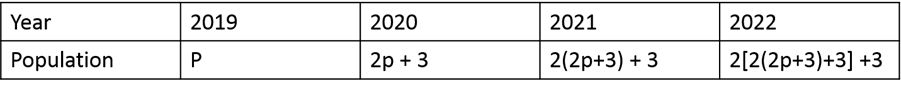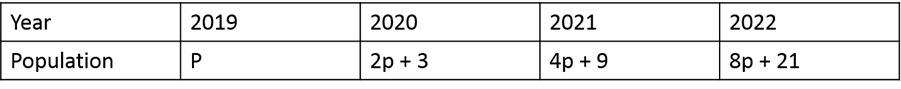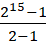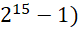# CAT 2019 Question Paper | Quants Slot 1

###### CAT Previous Year Paper | CAT Quants Questions | Question 10

This is a question where identifying which topic the question is from itself takes a minute. Of course this is from progressions. Usually there are a couple of questions around progressions in the CAT exam. This question tests a student's ability to convert the word problem into a mathematical problem post which is a straightforward application of the formulas . A good CAT Preparation would help you crack such questions.

Question 10 : If the population of a town is p in the beginning of any year then it becomes 3+2p in the beginning of the next year. If the population in the beginning of 2019 is 1000, then the population in the beginning of 2034 will be

1. (1003)15 + 6
2. (977)15 - 3
3. (1003)215 - 3
4. (977)214 + 3

## Best CAT Coaching in Chennai

#### CAT Coaching in Chennai - CAT 2022Limited Seats Available - Register Now!

From the given data,Simplifying,P increases in the series of 20P ,21P, 22P, 23P,... and so on
2034 is 15 years after 2019. So, the first part of the value would be 215 p
Now, let us observe the second pattern.
In 2021, the value increases to 2(2p + 3) + 3
On expanding, (2 x 2) +(2 x 3) + 3 = 2 x 2 + 3(1 + 2)
In 2022, the value increases to 2[2(2p+3) +3]+3
On expanding, 2((2 x 2p) + (2 x 3) + 3) + 3
= (2 x 2 x 2 x p) + (2x 2 x 3) + (2 x 3) + 3
= (2 x 2 x 2 x p) + 3(20 + 21 + 22)
In 2034, the value of the second part would be 3 (20 + 21 + 22 +.... +214 )
On simplifying using Geometric progression we get, 3 () = 3 (Total population = 215p +3 (Substituting p = 1000,
Total population = (215 x 1003) - 3

The question is "If the population of a town is p in the beginning of any year then it becomes 3+2p in the beginning of the next year. If the population in the beginning of 2019 is 1000, then the population in the beginning of 2034 will be"

##### Hence, the answer is (1003)215 - 3

Choice C is the correct answer.

###### CAT Coaching in ChennaiCAT 2023

Classroom Batches Starting Now! @Gopalapuram

###### Best CAT Coaching in Chennai Introductory offer of 5000/-

Attend a Demo Class

##### Where is 2IIM located?

2IIM Online CAT Coaching
A Fermat Education Initiative,
58/16, Indira Gandhi Street,
Kaveri Rangan Nagar, Saligramam, Chennai 600 093

##### How to reach 2IIM?

Mobile: (91) 99626 48484 / 94459 38484
WhatsApp: WhatsApp Now
Email: info@2iim.com